Monday 18th January 2021

CBSE Guess > Papers > Question Papers > Class XII > 2003 > Mathematics > Delhi Set -III

MATHEMATICS—2003 (Set III—Delhi)

Note: Except for the following questions, all the remaning questions have been asked in Set I, Set II .D. B., 2003.

SECTION - A

Q. 3. Ifshow thatQ. 8. Evaluate: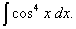Q. 13. In a correlation analysis, the following values are obtained about the variables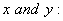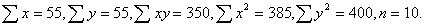Predict the value of y when the value of x is 6.

Q. 15. Prove that: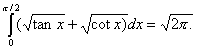Q. 16. Using matrix method, solve the following equations:SECTION - B

Q. 20. Three forces P, Q and R acting at Q, the circumcentre of a triangle ABC, along OA, OB and OC are in equilibrium. Show that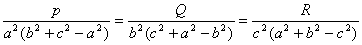Q. 25. Solve the following differentia equation:SECTION - C

Q. 20. Calculate the first four moments of the following data about 5:
5, 7, 9, 3, 4

Q. 22. A merchant in London buys dollars when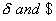rate isand sells when it falls to. If he makes a profit of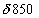in the transaction, find his original investment.

Q. 23. A, B, C and D entered into a partnership. A, B, C respectively subscribedpart of the capital and rest was subscribed by D. D is working partner and gets 4% of the total profit for that. The rest is divided amongst A, B, C and D in the ratio of their capitals. Find the shares of A, B. C and D in a total annual profit of Rs. 10,00,000.

 Mathematics 2003 Question Papers Class XII Delhi Outside Delhi Compartment Delhi Compartment Outside DelhiSet ISet ISet ISet ISet IISet IISet IISet IISet IIISet III

CBSE 2003 Question Papers Class XII